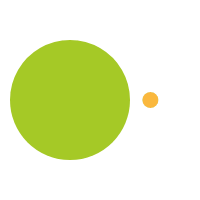June 23, 2016

# Number Nine, Fantastic Patterns in Multiples of Nine

9 x 0 = 00

9 x 1 = 09

9 x 2 = 18

9 x 3 = 27

9 x 4 = 36

9 x 5 = 45

9 x 6 = 54

9 x 7 = 63

9 x 8 = 72

9 x 9 = 81

9 x 10 = 90

## Nine Patterns

• Look at the tens place in each answer. It goes 0, 1, 2, 3, 4, 5, 6, 7, 8, 9
• Look at the ones place in each answer . It looks like 9, 8, 7, 6, 5, 4, 3, 2, 1, 0
• Notice that the tens digit in the answer is one less than the number 9 is being multiplied with (9 x 6 = 54) in 54, the tens digit 5 is one less than 6.
• Notice that if I draw a line between 9 x 5 = 45 and 9 x 6 - 54 that one side of answers is a mirror of the other side. 09, 18, 27, 36, 45 | 54, 63, 72, 81, 90
• Notice that in each answer if you add the two digit together that it equals 9. 1 + 8 = 9, 2 + 7 = 9, 3 + 6 = 9, 4 + 5 = 9. Infact if you add up the digits of any number down to a single digit it will equal 9. If I wanted to know if 247,356 was divisible by 9 I can add up all the digits, 2 + 4 + 7 + 3 + 5 + 6 = 27, 2 + 7 = 9. I know that 247,356 is divisible by 9.
• There is a finger trick as well, if you are multiplying 3 x 9, if i put the third finger down and look at my hands i will have two fingers up on one side of my hand and 7 on the other. I don't recommend this way of thinking about the patterns of 9 because it doesn't have the students thinking about the numbers.

• Students can also replace 9 with 10 and think 10 x 8 is 80 minus 8 = 72 or 10 x 5 = 50 - 5 = 45.
• Students can also think about adding 10 and subtracting 1. So we start with 9 x 1 = 9 then 9 x 2 = 18 or 9 + 10 - 1 = 18, 18 + 10 - 1 = 27

When I work with kids on the patterns I will sometimes put two strategies together that they can use to come up with the answer. If the problem is 9 x 8, I will say what is one less than 8, 7, and what plus 7 = 9, 2.